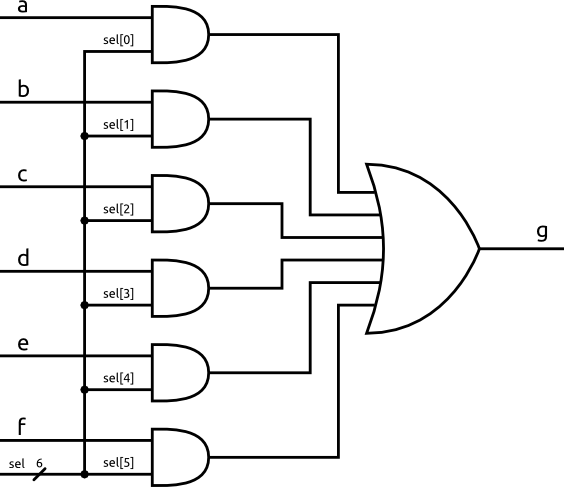# Multiplexers

A multiplexer is a circuit that allows you to select one of many inputs to be it's output. The output mirrors the selected input, so when that input is 0 the output is 0, when it is 1 the output is 1.

Multiplexers are often shown like thisOne of the inputs a through f is selected to be passed on through the output g by the sel input. In this case, the sel input is six bits wide and is one-hot encoded. That means when sel is 000001 input a is selected, while 000100 selects input c.

When you draw out circuits you should always use the block shown above as it is very clear what the circuit is doing and it is compact. However, it is important to understand how a multiplexer works. There are actually many ways to implement a multiplexer, each with their own trade offs. The circuit below is one of the more basic implementations.The input sel is split into it's six bits, each being fed into a different and gate. If an input's corresponding sel bit is 0 than the input is blocked from the output, however, when the sel bit is 1 then the output of the and gate mirrors the input. Since only the selected and gate can be 1 at any time the output g is the same as the selected and gate.

This circuit is very useful when you want to implement some conditional logic, like if X do this, else if Y do this, else do this. You just have to design a circuit that outputs the correct one-hot code for each condition to select the output you want.